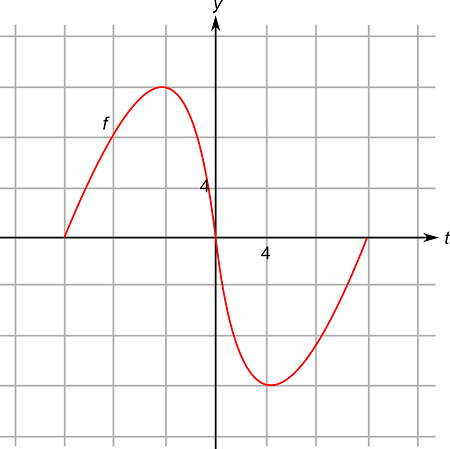# Let {eq}g\left( x \right) = \int\limits_{ - 12}^x {f\left( t \right)dt} {/eq}, where {eq}f {/eq} is the function whose graph is shown. Evaluate {eq}g\left( {-4} \right) {/eq}.## Question:

Let {eq}g\left( x \right) = \int\limits_{ - 12}^x {f\left( t \right)dt} {/eq}, where {eq}f {/eq} is the function whose graph is shown. Evaluate {eq}g\left( {-4} \right) {/eq}.## Integrability:

The integrability of a function is nothing but whether the given function is integrable in the given interval or not. If a function cannot be integrated into the given integral, the function is said to be a non-integrable function.

Given

• Consider the given graph of {eq}f {/eq}. And, {eq}g\left( x \right) = \int\limits_{ - 12}^x {f\left( t \right)dt} {/eq} .

The objective is to evaluate the value of {eq}g\left( { - 4} \right) {/eq}.

Consider the given integral,

{eq}\begin{align*} g\left( x \right) &= \int\limits_{ - 12}^{ - 4} {f\left( t \right)} dt\\ g\left( { - 4} \right) &= \int\limits_{ - 12}^{ - 4} {f\left( { - 4} \right)} dx\\ &= \int\limits_{ - 12}^{ - 4} {12dx} \\ &= \left[ {12x} \right]_{ - 12}^{ - 4} \end{align*} {/eq}

Simplifying further, we get

{eq}\begin{align*} g\left( { - 4} \right) &= \left[ {12\left( { - 4} \right) - 12\left( { - 12} \right)} \right]\\ &= - 48 + 144\\ &= 96 \end{align*} {/eq}

{eq}g\left( { - 4} \right) = 96 {/eq}